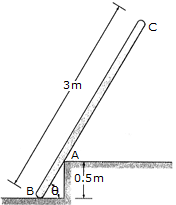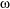Engineering Mechanics - PKRB: Impulse and Momentum - Discussion

Discussion :: PKRB: Impulse and Momentum - General Questions (Q.No.4)

4.The uniform pole has a mass of 15 kg and falls from rest when= 90° until it strikes the edge at A,= 60°. If the pole then begins to pivot about this point after contact, determine the pole's angular velocity just after the impact. Assume that the pole does not slip at B as it falls until it strikes A.

 [A].3 = 1.146 rad/s [B].3 = 0.537 rad/s [C].3 = 2.15 rad/s [D].3 = 1.528 rad/s

Explanation:

No answer description available for this question.

 Zahir said: (Apr 16, 2015) If time is not given how you can calculate frequency?

 Bhushan said: (Nov 25, 2015) Given data:mass = 15 kg.